# Which

Which decimals when subtracted equal 3.89:

a - b = 3.89

a =  10
b =  6.11

### Step-by-step explanation:

$a=10=10$
$b=a-3.89=10-3.89=6.11$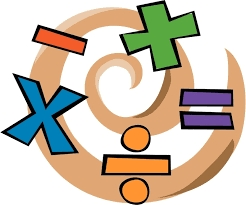Did you find an error or inaccuracy? Feel free to write us. Thank you!Tips to related online calculators
Do you have a linear equation or system of equations and looking for its solution? Or do you have a quadratic equation?

## Related math problems and questions:

• When 5When 5 is subtracted from both my numerator and denominator I become 3/4. What fraction i am?
• Complicated sum minus productWhat must be subtracted from the sum of 3/8 and 5/16 to get difference equal to the product of 5/8 and 3/16?
• The sum 10The sum of three decimals are 938.629. Two of them are 456.54 and 392.69. Find the third one.
• RootThe root of the equation (x-19)2 -10 = x2 -11x is (equal or greater or less than zero)? ...
• The two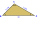The two angles of a triangle are 78° and 82°. So what is the measure of the remaining third angle?
• TranslateTranslate the following mathematical statement into an algebraic expression or equation. 1. Nine less that the quotient of a number and 3 2. The ratio of 3 and the sum of 4 and an unknown number 3. Eighty is the product of 4 and g 4. The sum of x and 18 i
• Two annulusesThe area of the annular circle formed by two circles with a common center is 100 cm2. The radius of the outer circle is equal to twice the radius of the inner circle. Determine the outside circle radius in centimeters.
• Unknown number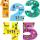Determine the unknown number, which double of its fourth square is equal the fifth its square.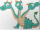Three sides of a quadrilateral are equal to the foreside which is 16 cm long. What is the length of the one with equal sides if the perimeter is 58 cm?
• Two thirdsFind two-thirds of the number equal to two-thirds of 99
• Expressions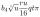Expression (3a-7).(3a+7) is identical with: ?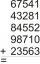Fill letters instead of digits so the indicated sum (equal letters represent equal digits). What number is hidden under the letter J? A A H A H O A H O J -------------------------- 4 3 2 1The sum of two numbers is 1. Identify this two numbers if you know that the half of first is equal to the third of second number.A number, X, is subtracted from 8 1/4. The result is 12 3/5. What is the value of X?Mrs. Petrová's garden had the shape of a square with a side length of 15 m. After its enlargement by 64 m2 (square), it had the shape of a square again. How many meters has the length of each side of the garden been extended?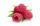Dano had 20 raspberries. John had 90% more raspberries than Dano. Determine how many raspberries have Dano and Juraj together.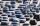The truck left Kremnica at 11:00 h at a speed of 60km/h. At 12:30 h, the passenger car started at an average speed of 80km/h. How many kilometers from Kremnica will the passenger car reach the truck, and when?